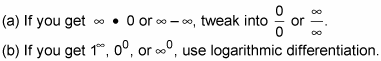##### Calculus Essentials For DummiesThe mathematics of limits underlies all of calculus. Limits sort of enable you to zoom in on the graph of a curve — further and further — until it becomes straight. Once it's straight, you can analyze the curve with regular-old algebra and geometry. That's the magic of calculus in a very small nutshell.

Here are some important things to remember when evaluating limits:

• The limit at a hole is the height of the hole.

• The limit at infinity is the height of the horizontal asymptote.

• Before trying other techniques, plug in the arrow number. If the result is:

• A number, you're done.

• A number over zero or infinity over zero, the answer is infinity.

• A number over infinity, the answer is zero.

• 0/0 or ∞/∞, use L'Hôpital's Rule.